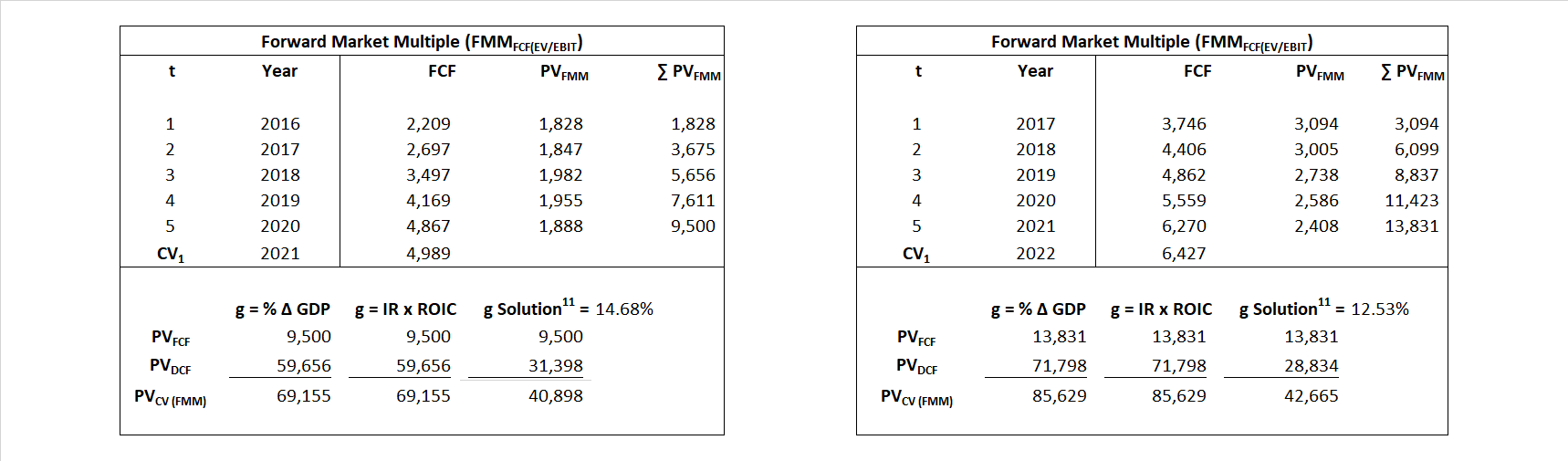# PayPal

## Analyst Listing

The following analysts provide coverage for the subject firm as of May 2016:

 Broker Analyst Analyst Email BMO Capital Markets Paulo Ribeiro paulo.ribeiro@bmo.com Nomura Instinet Bill Carcache bill.carcache@instinet.com Monness Crespi Hardt Cengiz M. Cakmak jcakmak@mchny.com Jefferies Ramsey El-Assal ramsey.elassal@jefferies.com Keefe Bruyette & Woods Sanjay Sakhrani ssakhrani@kbw.com Stifel Nicolaus Scott W. Devitt swdevitt@stifel.com Evercore ISI David Togut david.togut@evercoreisi.com Pacific Crest Securities-KBCM Josh Beck jbeck@pacific-crest.com Susquehanna Financial Group James Friedman james.friedman@sig.com Guggenheim Securities Eric Wasserstrom eric.wasserstrom@guggenheimpartners.com First Analysis Securities Corp Lawrence Berlin lberlin@firstanalysis.com Bernstein Research Lisa D. Ellis lisa.ellis@bernstein.com FBN Securities Shelby Seyrafi sseyrafi@fbnsecurities.com Deutsche Bank Research Bryan Keane bryan.keane@db.com William Blair Robert Napoli bnapoli@williamblair.com Raymond James Wayne Johnson wayne.johnson@raymondjames.com Canaccord Genuity Michael Graham mgraham@canaccordgenuity.com Stephens Inc Brett Huff brett.huff@stephens.com Needham Mayank Tandon mtandon@needhamco.com Oppenheimer Glenn Greene glenn.greene@opco.com Piper Jaffray Jason Deleeuw jason.s.deleeuw@pjc.com Wells Fargo Securities Timothy Willi timothy.willi@wellsfargo.com SunTrust Robinson Humphrey Andrew Jeffrey andrew.jeffrey@suntrust.com Cowen & Company Georgios Mihalos george.mihalos@cowen.com Loop Capital Markets Joseph A. Vafi joseph.vafi@loopcapital.com Atlantic Equities Christopher Hickey c.hickey@atlantic-equities.com Cantor Fitzgerald Naved Khan nkhan@cantor.com RBC Capital Markets Daniel Perlin daniel.perlin@rbccm.com BTIG Mark Palmer mpalmer@btig.com Compass Point Research John T. Williams jwilliams@compasspointllc.com Craig Hallum Capital Group Bradley Berning brad.berning@craig-hallum.com Wedbush Securities Moshe Katri moshe.katri@wedbush.com Credit Suisse Paul Condra paul.condra@credit-suisse.com

## Primary Input Data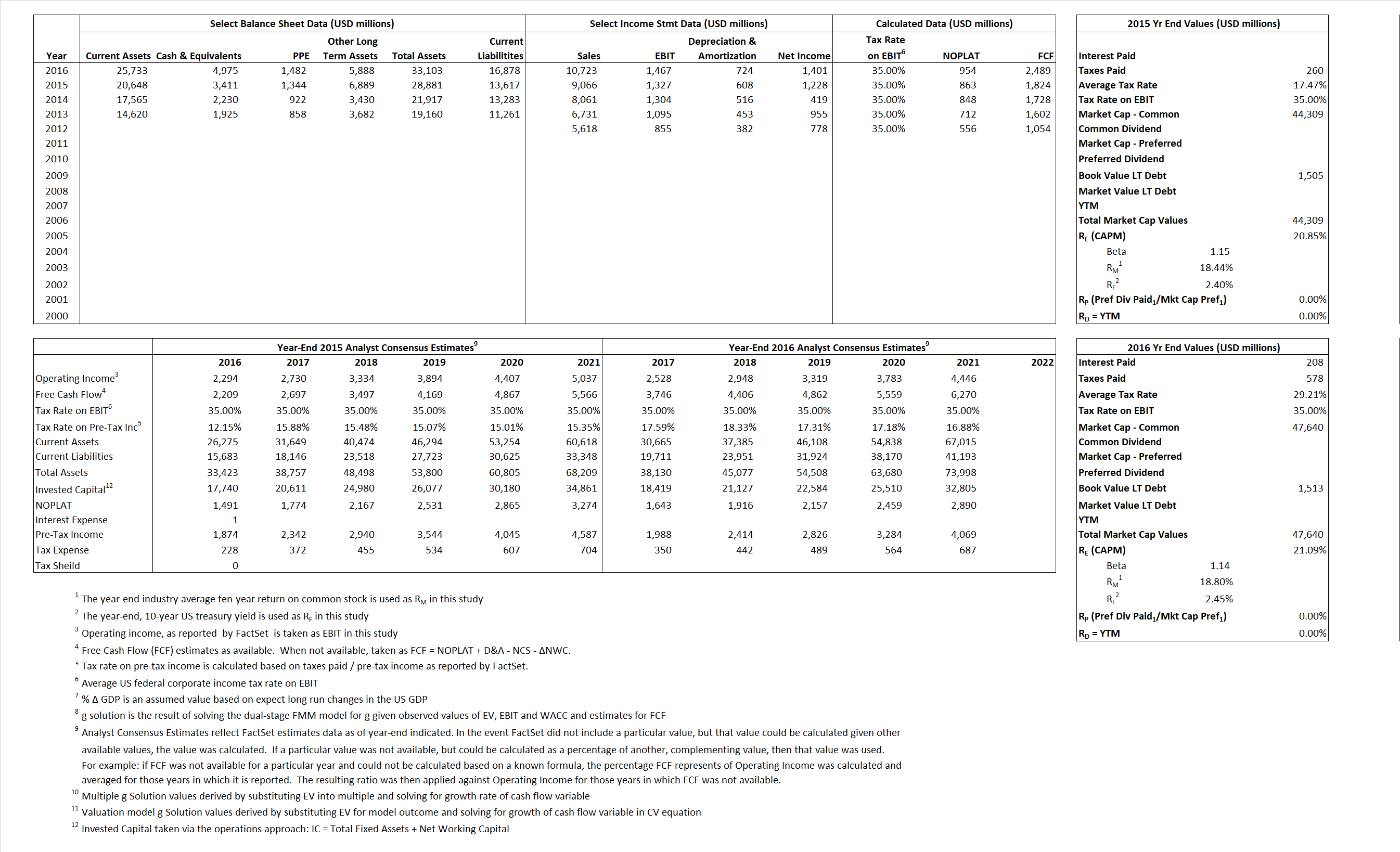## Derived Input Data

### Equational Form

Net Operating Profit Less Adjusted Taxes NOPLAT   863   954$NOPLAT\, =\, EBIT\, x\, (1 \,-\, Avg \,\,Tax\,\, Rate\,\, on\,\, EBIT)$
Free Cash Flow FCF  1,824  2,489$FCF\,=NOPLAT\,+\,Non-Cash\,Expenses-\Delta NWC\,-\,NCS$
Tax Shield TS 0  61$TS\,=\,Interest\,\,Paid\,\,x\,\, Avg \,\,Tax\,\,Rate\,\, on\,\, Pre-Tax\,\, Income$
Invested Capital IC  15,264  16,225$IC\,=\,Fixed\,\,Operating\,\,Assets\,\,+\,\,Net\,\, Working\,\, Capital$
Return on Invested Capital ROIC 5.65% 5.88%$ROIC\,=\,\frac { NOPLAT }{ IC }$
Net Investment NetInv  7,238  1,685$NetInv\,=\,{ {IC}_{1}}-{{IC}_{0}}+Depreciation$
Investment Rate IR 839.14% 176.71%$IR\,=\,\frac {NetInv}{NOPLAT}$
Weighted Average Cost of Capital WACCMarket 20.85% 21.09%$WACC\,=\,\frac { E }{ V } { R }_{ E }\,+\,\frac { P }{ V } { R }_{ P }\,+\,\frac { D }{ V } { R }_{ D }\left( 1- Avg\,\, Tax\,\,Rate\,\,on\,\,Pre-Tax\,\,Income \right)$
WACCBook  10.46% 9.91%
Enterprise value EVMarket  40,898  42,665$EV\,=\,Market\,\,Cap\,\,Equity\,+\,\,Long\,\,Term\,\,Debt\,-\,Cash$
EVBook  40,839  44,178
EV/EBIT Multiple$\frac{EV_{Market}}{EBIT}$  30.82  29.08$EV/EBIT\,=\,\frac { EV}{ EBIT}$
Long-Run Growth g = IR x ROIC
47.42% 10.39% Long-run growth rates of the income variable  are used in the Continuing Value portion of the valuation models.
g = %$\Delta$ GDP  2.50% 2.50%

## Valuation Model Outcomes

The outcomes presented in this study are the result of original input data, derived data, and synthesized inputs and, depending on the equational form of any particular valuation model, may result in irrelevant or implausible results.  For example, in the event WACC < g, the value of this term, often found in the denominator of an equation’s continuation value term, will be expressly negative and may result in a negative overall valuation for the firm.  In the event of a WACC < g relation, the model form as applied to the subject firm offers an irrelevant outcome.

### Equational form

Key Value Driver (NOPLAT) KVD (NOPLAT)${ Value }_{ DCF/KVD }=\sum { \frac { NOPLAT_{ t } }{ { \left( 1+WACC \right) }^{ t } } +\frac { \frac { { NOPLAT }_{ 1 }\left( 1-\frac { g }{ ROIC } \right) }{ WACC-g } }{ { \left( 1+WACC \right) }^{ t } } }$Key Value Driver (FCF) KVD (FCF)${ Value }_{ DCF/KVD }=\sum { \frac { FCF_{ t } }{ { \left( 1+WACC \right) }^{ t } } +\frac { \frac { { NOPLAT }_{ 1 }\left( 1-\frac { g }{ ROIC } \right) }{ WACC-g } }{ { \left( 1+WACC \right) }^{ t } } }$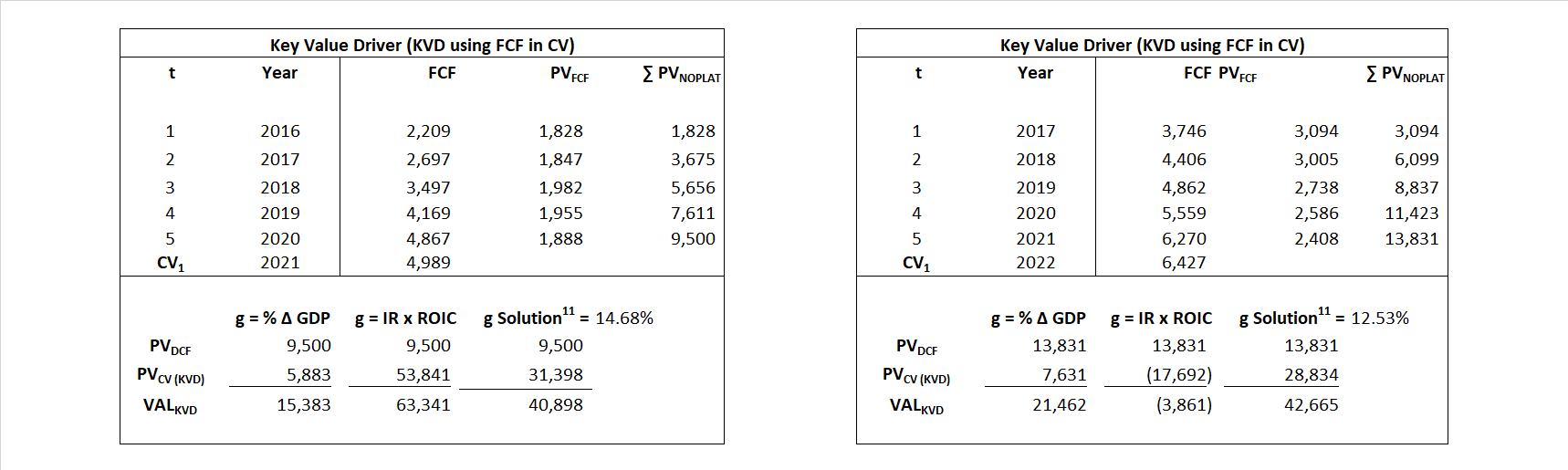Free Cash Flow FCF${ Value }_{ DCF/FCF }=\sum { \frac { FCF_{ t } }{ { \left( 1+WACC \right) }^{ t } } +\frac { \frac { { FCF }_{ 1 }}{ WACC-g } }{ { \left( 1+WACC \right) }^{ t } } }$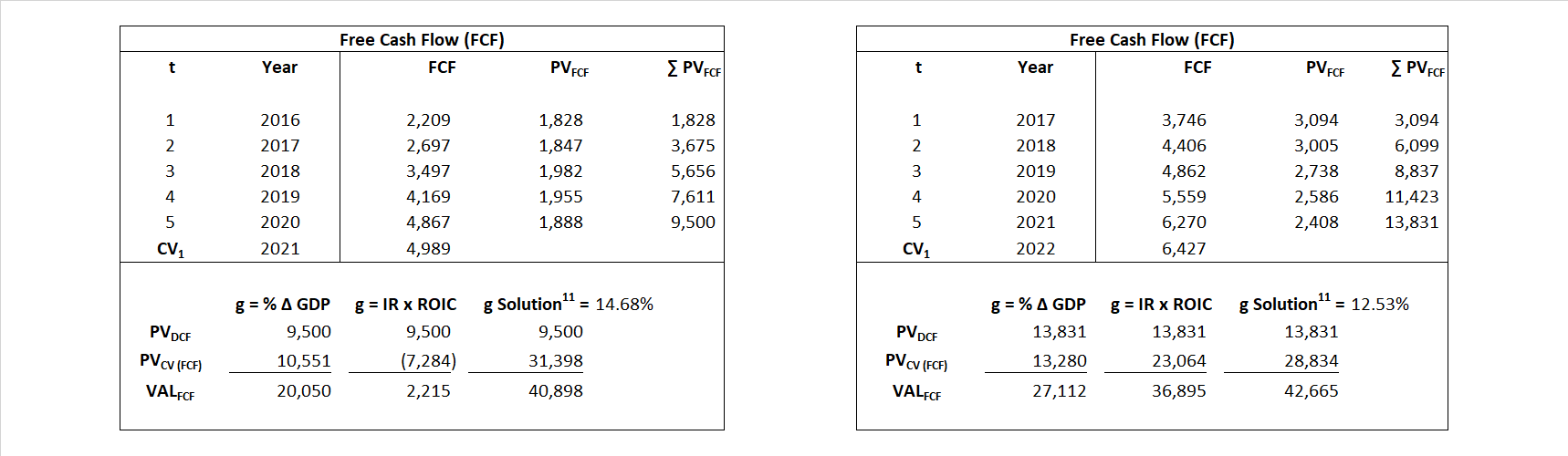Economic Profit ECON π${ Value }_{ { ECON\pi } }= I{ C }_{ 0 }+\sum { \frac { { IC }_{ t-1 }(ROI{ C }_{t}-WAC{C}_{t}) }{ { \left( 1+WACC \right) }^{ t } }+ \frac {\frac { I{C}_{0}\ x\ (ROI{C}_{1}\ -\ WAC{C}_{1}) }{ WACC-g } }{ { \left( 1+WACC \right) }^{ t } } }$Adjusted Present Value APV${ Value }_{ APV }=\sum { \frac { FCF_{ t } }{ { \left( 1+{ k }_{ u } \right) }^{ t } } +\frac { \frac { { FCF }_{ 1 }}{ { k }_{ u }-g } }{ { \left( 1+{ k }_{ u } \right) }^{ t } } } +\sum { \frac { { TS }_{ t } }{ { \left( 1+{ k }_{ tax } \right) }^{ t } } +\frac { \frac { { TS }_{ 1 }}{ { k }_{ tax }-g } }{ { \left( 1+{ k }_{ tax } \right) }^{ t } } }$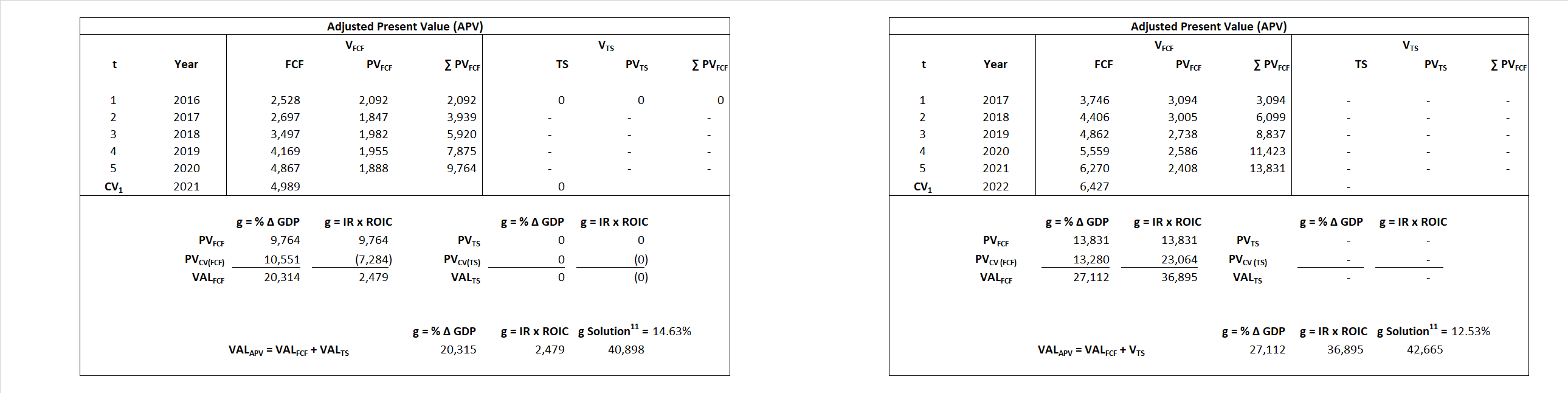Forward Market Multiple FMM${ Value }_{ DCF/FMM}=\sum { \frac { FCF_{ t } }{ { \left( 1+WACC \right) }^{ t } } +\frac { { EBIT }_{ 1 }\,{x}\,{FMM}}{ { \left( 1+WACC \right) }^{ t } } }{\,\,\,; \,\,FMM\,=\,\frac{{EV}_{t=0}}{{EBIT}_{t=0}}}$